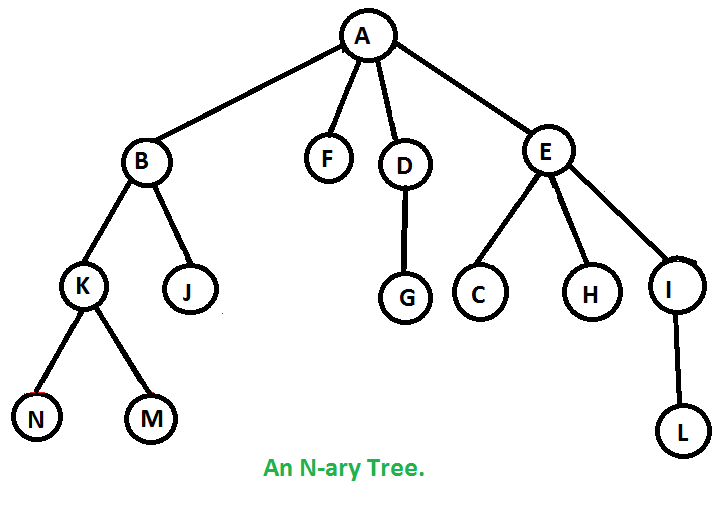# Preorder Traversal of N-ary Tree Without Recursion

Given an n-ary tree, print preorder traversal of it.

Example :

Preorder traversal of below tree is A B K N M J F D G E C H I L## Recommended: Please try your approach on {IDE} first, before moving on to the solution.

The idea is to use stack like iterative preorder traversal of binary tree.

1) Create an empty stack to store nodes.
2) Push the root node to the stack.
3) Run a loop while the stack is not empty
….a) Pop the top node from stack.
….b) Print the popped node.
….c) Store all the children of popped node onto the stack. We must store children from right to left so that leftmost node is popped first.
4) If stack is empty then we are done.

## C++

 `// C++ program to traverse an N-ary tree ` `// without recursion ` `#include ` `using` `namespace` `std; ` ` `  `// Structure of a node of an n-ary tree ` `struct` `Node { ` `    ``char` `key; ` `    ``vector child; ` `}; ` ` `  `// Utility function to create a new tree node ` `Node* newNode(``int` `key) ` `{ ` `    ``Node* temp = ``new` `Node; ` `    ``temp->key = key; ` `    ``return` `temp; ` `} ` ` `  `// Function to traverse tree without recursion ` `void` `traverse_tree(``struct` `Node* root) ` `{ ` `    ``// Stack to store the nodes ` `    ``stack nodes; ` ` `  `    ``// push the current node onto the stack ` `    ``nodes.push(root); ` ` `  `    ``// loop while the stack is not empty ` `    ``while` `(!nodes.empty()) { ` ` `  `        ``// store the current node and pop it from the stack ` `        ``Node* curr = nodes.top(); ` `        ``nodes.pop(); ` ` `  `        ``// current node has been travarsed ` `     ``if``(curr != NULL) ` `      ``{ ` `         ``cout << curr->key << ``" "``; ` ` `  `        ``// store all the childrent of current node from ` `        ``// right to left. ` `        ``vector::iterator it = curr->child.end(); ` ` `  `        ``while` `(it != curr->child.begin()) { ` `            ``it--; ` `            ``nodes.push(*it); ` `        ``} ` `      ``} ` `    ``} ` `} ` `// Driver program ` `int` `main() ` `{ ` `    ``/*   Let us create below tree  ` `   ``*            A  ` `   ``*        /  / \  \  ` `   ``*       B  F   D  E  ` `   ``*      / \     |  /|\  ` `   ``*     K  J     G C H I  ` `   ``*    / \         |   |  ` `   ``*   N   M        O   L  ` `   ``*/` ` `  `    ``Node* root = newNode(``'A'``); ` `    ``(root->child).push_back(newNode(``'B'``)); ` `    ``(root->child).push_back(newNode(``'F'``)); ` `    ``(root->child).push_back(newNode(``'D'``)); ` `    ``(root->child).push_back(newNode(``'E'``)); ` `    ``(root->child->child).push_back(newNode(``'K'``)); ` `    ``(root->child->child).push_back(newNode(``'J'``)); ` `    ``(root->child->child).push_back(newNode(``'G'``)); ` `    ``(root->child->child).push_back(newNode(``'C'``)); ` `    ``(root->child->child).push_back(newNode(``'H'``)); ` `    ``(root->child->child).push_back(newNode(``'I'``)); ` `    ``(root->child->child->child).push_back(newNode(``'N'``)); ` `    ``(root->child->child->child).push_back(newNode(``'M'``)); ` `    ``(root->child->child->child).push_back(newNode(``'O'``)); ` `    ``(root->child->child->child).push_back(newNode(``'L'``)); ` ` `  `    ``traverse_tree(root); ` ` `  `    ``return` `0; ` `} `

## Python3

 `#Python program to find height of full binary tree  ` `# using preorder ` ` `  `class` `newNode():  ` `   `  `    ``def` `__init__(``self``, key):  ` `        ``self``.key ``=` `key ` `        ``# all children are stored in a list  ` `        ``self``.child ``=``[] ` `         `  `# Function to traverse tree without recursion  ` `def` `traverse_tree(root): ` `  `  `    ``# Stack to store the nodes  ` `    ``nodes``=``[] ` ` `  `    ``# push the current node onto the stack  ` `    ``nodes.append(root)  ` `   `  `    ``# loop while the stack is not empty  ` `    ``while` `(``len``(nodes)):   ` `   `  `        ``# store the current node and pop it from the stack  ` `        ``curr ``=` `nodes[``0``]  ` `        ``nodes.pop(``0``)  ` `   `  `        ``# current node has been travarsed  ` `        ``print``(curr.key,end``=``" "``) ` `        ``# store all the childrent of current node from  ` `        ``# right to left.  ` `        ``for` `it ``in` `range``(``len``(curr.child)``-``1``,``-``1``,``-``1``):   ` `            ``nodes.insert(``0``,curr.child[it]) ` `  `  `          `  `# Driver program to test above functions  ` `if` `__name__ ``=``=` `'__main__'``: ` `    ``"""   Let us create below tree   ` `   ``*            A   ` `   ``*        /  / \  \   ` `   ``*       B  F   D  E   ` `   ``*      / \     |  /|\   ` `   ``*     K  J     G C H I   ` `   ``*    / \         |   |   ` `   ``*   N   M        O   L   ` `   ``"""` `    ``root ``=` `newNode(``'A'``)  ` `    ``(root.child).append(newNode(``'B'``))  ` `    ``(root.child).append(newNode(``'F'``))  ` `    ``(root.child).append(newNode(``'D'``))  ` `    ``(root.child).append(newNode(``'E'``))  ` `    ``(root.child[``0``].child).append(newNode(``'K'``))  ` `    ``(root.child[``0``].child).append(newNode(``'J'``))  ` `    ``(root.child[``2``].child).append(newNode(``'G'``))  ` `    ``(root.child[``3``].child).append(newNode(``'C'``))  ` `    ``(root.child[``3``].child).append(newNode(``'H'``))  ` `    ``(root.child[``3``].child).append(newNode(``'I'``))  ` `    ``(root.child[``0``].child[``0``].child).append(newNode(``'N'``))  ` `    ``(root.child[``0``].child[``0``].child).append(newNode(``'M'``))  ` `    ``(root.child[``3``].child[``0``].child).append(newNode(``'O'``))  ` `    ``(root.child[``3``].child[``2``].child).append(newNode(``'L'``))  ` `   `  `    ``traverse_tree(root) ` `  `  `# This code is contributed by SHUBHAMSINGH10 `

Output:

```A B K N M J F D G E C O H I L
```

GeeksforGeeks has prepared a complete interview preparation course with premium videos, theory, practice problems, TA support and many more features. Please refer Placement 100 for details

My Personal Notes arrow_drop_upCheck out this Author's contributed articles.

If you like GeeksforGeeks and would like to contribute, you can also write an article using contribute.geeksforgeeks.org or mail your article to contribute@geeksforgeeks.org. See your article appearing on the GeeksforGeeks main page and help other Geeks.

Please Improve this article if you find anything incorrect by clicking on the "Improve Article" button below.

Improved By : SHUBHAMSINGH10, Enigma341

Article Tags :
Practice Tags :

1

Please write to us at contribute@geeksforgeeks.org to report any issue with the above content.